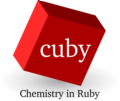back to Interfaces

# Mixer interface

This virtual interface mixes two calculations (potentials) in a specified ratio (mixer_a_to_b ratio). The two calculations defined independently in two subsections of the input file named calculation_a and calculation_b. These two calculations are run independently and the resulting energy and gradient is a linear combination of their results.

The first possible use is to mix two different potentials for the same system by defining different calculations to be used.

The second possibility is to use the same method for the calculation and change elements of some atoms using keyword mixer_transmutations.

## Example

Optimization of Halogen anion ... water complex where the halogen atom is 1:1 mixture of Br and Cl.

Input file:

``````interface: mixer
charge: -1

mixer_ratio_a_to_b: 0.5

calculation_a:
interface: metainterface
metainterface: pm6

calculation_b:
# Use the same calculation setup, change first atom to chlorine
interface: metainterface
metainterface: pm6
mixer_transmutations:
1: Cl

restart_file: restart.xyz
opt_quality: 0.1
``````

Geometry:

``````4

Br    0.000000000   0.000000000   0.000000000
H    3.000000000   0.000000000   0.000000000
O    3.999847695   0.017452406   0.000000000
H    4.341865325  -0.922096229   0.016501574``````

## Keywords

The interface requires two subsections in the input:

• calculation_a
• calculation_b